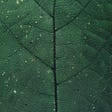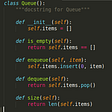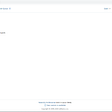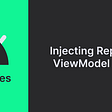# We are too mature to make a ViewGroup 😉

`<?xml version="1.0" encoding="utf-8"?><androidx.constraintlayout.widget.ConstraintLayout    xmlns:android="http://schemas.android.com/apk/res/android"    xmlns:app="http://schemas.android.com/apk/res-auto"    android:layout_width="match_parent"    android:layout_height="match_parent">    <com.google.android.material.floatingactionbutton.FloatingActionButton        android:id="@+id/fab"        android:layout_width="wrap_content"        android:layout_height="wrap_content"        app:fabSize="normal"        app:backgroundTint="@color/colorPrimary"        app:layout_constraintStart_toStartOf="parent"        app:layout_constraintTop_toTopOf="parent"        app:layout_constraintEnd_toEndOf="parent"        app:layout_constraintBottom_toBottomOf="parent"/>    <TextView        android:layout_width="18sp"        android:layout_height="18sp"        android:background="@drawable/bg_round"        android:elevation="6dp"        android:gravity="center"        android:text="9"        android:textColor="#fff"        android:textSize="10sp"        app:layout_constraintTop_toTopOf="@id/fab"        app:layout_constraintEnd_toEndOf="@id/fab"/></androidx.constraintlayout.widget.ConstraintLayout>`

# Make it right then!

`private val textPaint = TextPaint(ANTI_ALIAS_FLAG).apply {    textAlign = Paint.Align.LEFT}private val tintPaint = Paint(ANTI_ALIAS_FLAG)`
`var counterTextColor: Int    get() = textPaint.color    set(value) {        val was = textPaint.color        if (was != value) {            textPaint.color = value            invalidate()        }    }var counterTint: Int    get() = tintPaint.color    set(value) {        val was = tintPaint.color        if (was != value) {            tintPaint.color = value            invalidate()        }    }var counterTextSize: Float    get() = textPaint.textSize    set(value) {        val was = textPaint.textSize        if (was != value) {            textPaint.textSize = value            invalidate()            requestLayout()        }    }var counterTypeface: Typeface?    get() = textPaint.typeface    set(value) {        val was = textPaint.typeface        if (was != value) {            textPaint.typeface = value            invalidate()            requestLayout()        }    }var counterTextPadding: Float = 0f    set(value) {        if (field != value) {            field = value            invalidate()            requestLayout()        }    }`
`var maxCount: Int = 9    set(@IntRange(from = 1) value) {        if (field != value) {            field = value            countMaxStr = "\$value+"            requestLayout()        }    }var count: Int = 0    set(@IntRange(from = 0) value) {        if (field != value) {            field = value            countStr = countStr(value)            textPaint.getTextBounds(countStr, counterTextBounds)            invalidate()        }    }private fun countStr(count: Int) = if (count > maxCount) "\$maxCount+" else count.toString()`
`private var fabBounds: Rect = Rect()private var counterBounds: RectF = RectF()private var counterTextBounds: Rect = Rect()private var counterMaxTextBounds: Rect = Rect()private var counterPossibleCenter: PointF = PointF()`

## Drawing

`override fun onDraw(canvas: Canvas) {    super.onDraw(canvas)    if (count > 0) {        canvas.drawCircle(counterBounds, tintPaint)        val textX = counterBounds.centerX() - counterTextBounds.width() / 2f - counterTextBounds.left        val textY = counterBounds.centerY() + counterTextBounds.height() / 2f - counterTextBounds.bottom        canvas.drawText(countStr, textX, textY, textPaint)    }}fun Canvas.drawCircle(bounds: RectF, paint: Paint) =        drawCircle(bounds.centerX(), bounds.centerY(), min(bounds.width(), bounds.height()) / 2, paint)`

## Measuring

`override fun onMeasure(widthMeasureSpec: Int, heightMeasureSpec: Int) {    super.onMeasure(widthMeasureSpec, heightMeasureSpec)    calculateCounterBounds(counterBounds)}`
`private fun calculateCounterCenter(radius: Float, outPoint: PointF) =        calculateCounterCenter(radius, (PI / 4).toFloat(), outPoint)private fun calculateCounterCenter(radius: Float, angle: Float, outPoint: PointF) {    outPoint.x = radius * cos(angle)    outPoint.y = radius * sin(angle)}`
`private fun calculateCounterCenter(inBounds: Rect, outPoint: PointF) {    val radius = min(inBounds.width(), inBounds.height()) / 2f    calculateCounterCenter(radius, outPoint)    outPoint.x = inBounds.centerX() + outPoint.x    outPoint.y = inBounds.centerY() - outPoint.y}`
`textPaint.getTextBounds(countMaxStr, counterMaxTextBounds)val counterDiameter = max(counterMaxTextBounds.width(), counterMaxTextBounds.height()) + 2 * counterTextPadding//wherefun Paint.getTextBounds(text: String, bounds: Rect) = getTextBounds(text, 0, text.length, bounds)`
`val counterRight = min(counterPossibleCenter.x + counterDiameter / 2, fabBounds.right.toFloat())val counterTop = max(counterPossibleCenter.y - counterDiameter / 2, fabBounds.top.toFloat())`
`private fun calculateCounterBounds(outRect: RectF) {    getMeasuredContentRect(fabBounds)    calculateCounterCenter(fabBounds, counterPossibleCenter)    textPaint.getTextBounds(countMaxStr, counterMaxTextBounds)    val counterDiameter = max(counterMaxTextBounds.width(), counterMaxTextBounds.height()) + 2 * counterTextPadding    val counterRight = min(counterPossibleCenter.x + counterDiameter / 2, fabBounds.right.toFloat())    val counterTop = max(counterPossibleCenter.y - counterDiameter / 2, fabBounds.top.toFloat())    outRect.set(counterRight - counterDiameter, counterTop, counterRight, counterTop + counterDiameter)}`

# Support `rtl` direction

`//in a calculateCounterBounds fun right after formulating outRectif (isRtl()) {    val newLeft = fabBounds.left + (fabBounds.right - outRect.right)    val newRight = fabBounds.left + (fabBounds.right - outRect.left)    outRect.left = newLeft    outRect.right = newRight}//whereprivate fun isRtl() = layoutDirection == View.LAYOUT_DIRECTION_RTL`

# Afterwords

--

--

--

## More from Michael Spitsin

Love being creative to solve some problems with an simple and elegant ways

Love podcasts or audiobooks? Learn on the go with our new app.

## Flutter vs React Native -What to choose?## An improved development workflow on Business Central using our new DevTools## A step by Step Guide to Install Tensorflow GPU on Ubuntu 18.04 LTS## Cars line-up at a car wash | Queue Data Structure## How to CI/CD in IOS using TeamCity## Michael Spitsin

Love being creative to solve some problems with an simple and elegant ways

## More from Medium